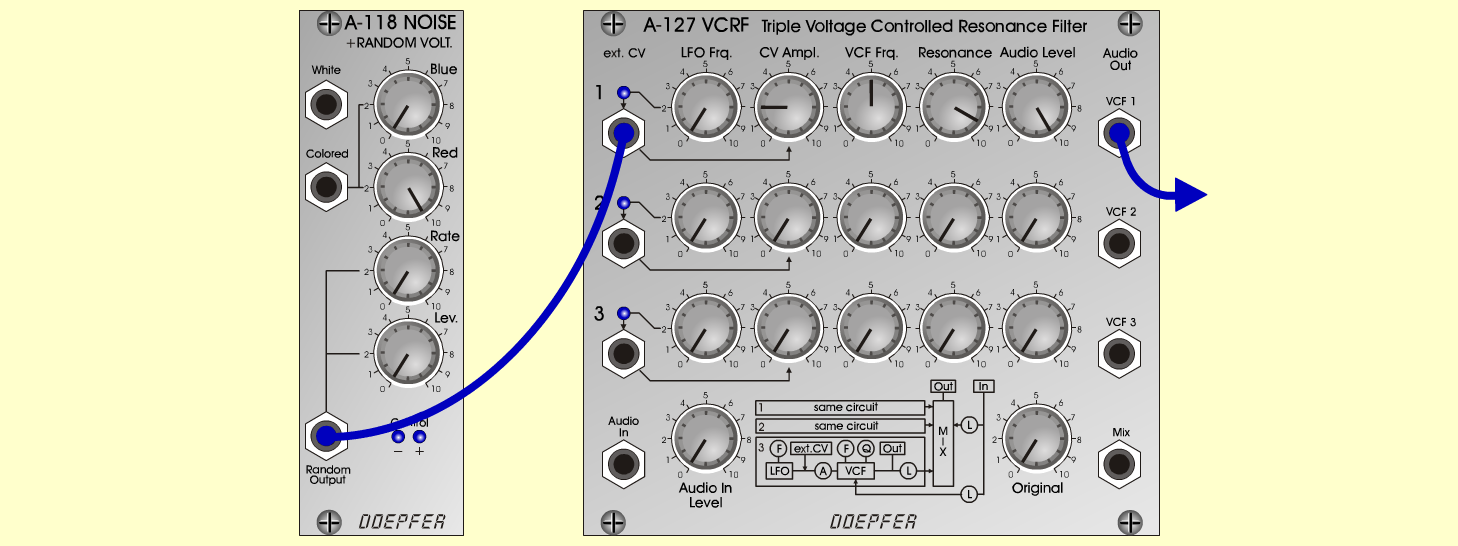Magic PotionFXD o e p f e r A - 100 Connections: Settings: A-118 (Random)  <=>  A-127 (ext. CV 1) A-127 (VCF 1)  <=> Amplifier A-118 (Blue = 0, Red = 10, Rate = 0, Level = 8) A-127 (Audio In = 0, Original = 0; Row 1: LFO Freq = 0, CV Ampl = 2, VCF Frq = 5, Res = 9, Audio Level = 10) Josef MuellerSound sample Magic Potion